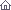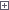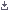Electro-Hobby Wednesday, 22.05.2019, 05:38 Circuits Welcome Guest | RSS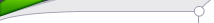Search

 StatisticsTotal online: 1 Guests: 1 Users: 0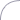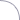Home » Tests and Measurement » 3 digits Digital Ammeter
 14:35 3 digits Digital Ammeter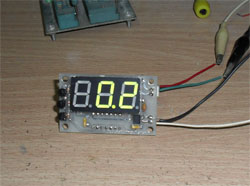Here is a digital Ammeter based on PIC16F684 and ACS712 current sensor. The measured DC or AC current will display on 3 digits 7-segment with resolution 100 mA. The current sensor in this project is ACS712ELCTR-30A-T from Allegro(I got from ebay). It can measure the AC or DC current up to 30A with 66 mV/A output sensitivity.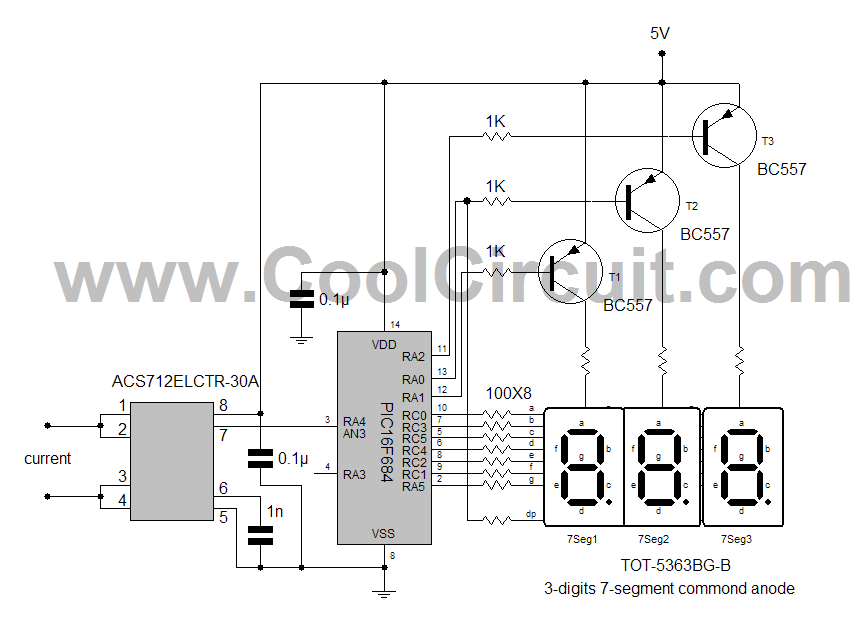The microcontroller PIC16F684 reading analog value from the ACS712 current sensor output and convert to curent for diplaying on 7-segment. All 7-segments are commond anode type and driven by PNP transistors. Actually, this circuit suitable for measuring DC current e.g. solar panel to battery, battery to load.Calculating volt to amp for Vcc 5VFrom ACS712 data sheet we know :0 A = Vcc/2 = 2.5V or 512 analog counts or 0x200. Sensitivity 66mV/A for 30A version. So Volt at 30A = 2.5V + (66mV/A x 30A) = 4.48V or 917.504 analog counts Volt at -30A = 2.5v - (66mV/A x 30A) = 0.52V or 106.5 analog counts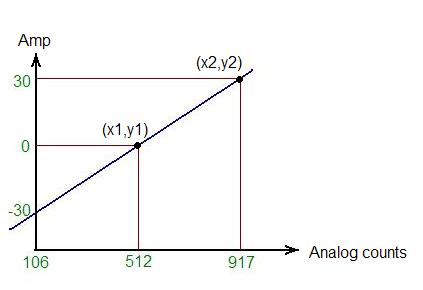With basic math linear equationy = mx + b ----------- Equ. 1 m = (y2 - y1) / (x2-x1) -------------Equ.2 So we get m = (30-0) / (917 - 512) = 0.074 substitute m in Equ. 1 at output 0A, we will get b 0 = 0.074*512 + b b = -37.888 thus, the final equation use in software is : A = 0.074*(analog_counts) - 37.888Example : if analog counts = 512 we get 0 Aif analog counts = 917 we get about +30Aif analog counts = 106 we get about -30A The PCB track and wire of the input current in this project is used for measuring small current(for learning). If you want to measure up to 30A the PCB track and wire of input current should be large enough.Schematic and firmware This is zipped file for picc source code, firmware and  schematic for this project Category: Tests and Measurement | Views: 4093 |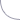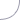Advertising

 Copyright MyCorp © 2019 Free web hosting — uCoz